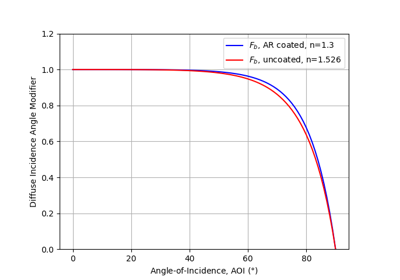pvlib.iam.physical¶

pvlib.iam.physical(aoi, n=1.526, K=4.0, L=0.002)[source]

Determine the incidence angle modifier using refractive index n, extinction coefficient K, and glazing thickness L.

iam.physical calculates the incidence angle modifier as described in 1, Section 3. The calculation is based on a physical model of absorbtion and transmission through a transparent cover.

Parameters
• aoi (numeric) – The angle of incidence between the module normal vector and the sun-beam vector in degrees. Angles of 0 are replaced with 1e-06 to ensure non-nan results. Angles of nan will result in nan.

• n (numeric, default 1.526) – The effective index of refraction (unitless). Reference 1 indicates that a value of 1.526 is acceptable for glass.

• K (numeric, default 4.0) – The glazing extinction coefficient in units of 1/meters. Reference  indicates that a value of 4 is reasonable for “water white” glass.

• L (numeric, default 0.002) – The glazing thickness in units of meters. Reference 1 indicates that 0.002 meters (2 mm) is reasonable for most glass-covered PV panels.

Returns

iam (numeric) – The incident angle modifier

Notes

The pvlib python authors believe that Eqn. 14 in 1 is incorrect, which presents $$\theta_{r} = \arcsin(n \sin(AOI))$$. Here, $$\theta_{r} = \arcsin(1/n \times \sin(AOI))$$

References

1(1,2,3,4)

W. De Soto et al., “Improvement and validation of a model for photovoltaic array performance”, Solar Energy, vol 80, pp. 78-88, 2006.

2

Duffie, John A. & Beckman, William A.. (2006). Solar Engineering of Thermal Processes, third edition. [Books24x7 version] Available from http://common.books24x7.com/toc.aspx?bookid=17160.

Examples using pvlib.iam.physical¶